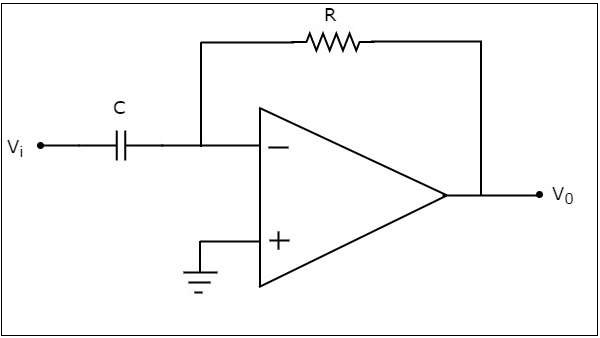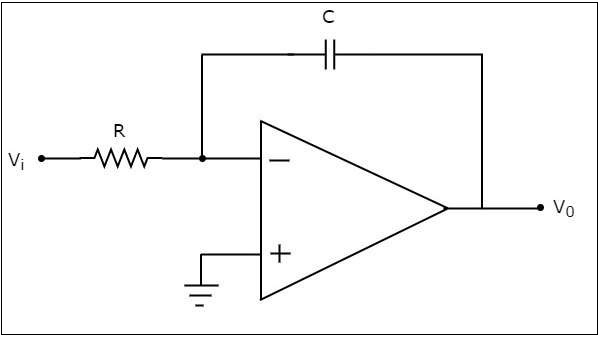# Differentiator And Integrator

The electronic circuits which perform the mathematical operations such as differentiation and integration are called as differentiator and integrator, respectively.

This chapter discusses in detail about op-amp based differentiator and integrator. Please note that these also come under linear applications of op-amp.

## Differentiator

A differentiator is an electronic circuit that produces an output equal to the first derivative of its input. This section discusses about the op-amp based differentiator in detail.

An op-amp based differentiator produces an output, which is equal to the differential of input voltage that is applied to its inverting terminal. The circuit diagram of an op-amp based differentiator is shown in the following figure −In the above circuit, the non-inverting input terminal of the op-amp is connected to ground. That means zero volts is applied to its non-inverting input terminal.

According to the virtual short concept, the voltage at the inverting input terminal of opamp will be equal to the voltage present at its non-inverting input terminal. So, the voltage at the inverting input terminal of op-amp will be zero volts.

The nodal equation at the inverting input terminal's node is −

$$C\frac{\text{d}(0-V_{i})}{\text{d}t}+\frac{0-V_0}{R}=0$$

$$=>-C\frac{\text{d}V_{i}}{\text{d}t}=\frac{V_0}{R}$$

$$=>V_{0}=-RC\frac{\text{d}V_{i}}{\text{d}t}$$

If $RC=1\sec$, then the output voltage $V_{0}$ will be −

$$V_{0}=-\frac{\text{d}V_{i}}{\text{d}t}$$

Thus, the op-amp based differentiator circuit shown above will produce an output, which is the differential of input voltage $V_{i}$, when the magnitudes of impedances of resistor and capacitor are reciprocal to each other.

Note that the output voltage $V_{0}$ is having a negative sign, which indicates that there exists a 1800 phase difference between the input and the output.

## Integrator

An integrator is an electronic circuit that produces an output that is the integration of the applied input. This section discusses about the op-amp based integrator.

An op-amp based integrator produces an output, which is an integral of the input voltage applied to its inverting terminal. The circuit diagram of an op-amp based integrator is shown in the following figure −In the circuit shown above, the non-inverting input terminal of the op-amp is connected to ground. That means zero volts is applied to its non-inverting input terminal.

According to virtual short concept, the voltage at the inverting input terminal of op-amp will be equal to the voltage present at its non-inverting input terminal. So, the voltage at the inverting input terminal of op-amp will be zero volts.

The nodal equation at the inverting input terminal is −

$$\frac{0-V_i}{R}+C\frac{\text{d}(0-V_{0})}{\text{d}t}=0$$

$$=>\frac{-V_i}{R}=C\frac{\text{d}V_{0}}{\text{d}t}$$

$$=>\frac{\text{d}V_{0}}{\text{d}t}=-\frac{V_i}{RC}$$

$$=>{d}V_{0}=\left(-\frac{V_i}{RC}\right){\text{d}t}$$

Integrating both sides of the equation shown above, we get −

$$\int{d}V_{0}=\int\left(-\frac{V_i}{RC}\right){\text{d}t}$$

$$=>V_{0}=-\frac{1}{RC}\int V_{t}{\text{d}t}$$

If $RC=1\sec$, then the output voltage, $V_{0}$ will be −

$$V_{0}=-\int V_{i}{\text{d}t}$$

So, the op-amp based integrator circuit discussed above will produce an output, which is the integral of input voltage $V_{i}$, when the magnitude of impedances of resistor and capacitor are reciprocal to each other.

Note − The output voltage, $V_{0}$ is having a negative sign, which indicates that there exists 1800 phase difference between the input and the output.